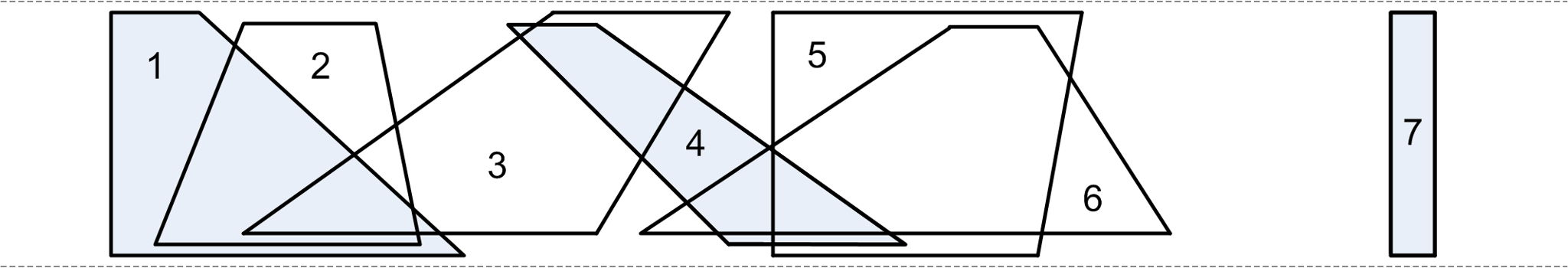시간 제한 메모리 제한 제출 정답 맞은 사람 정답 비율
1 초 128 MB 49 31 24 61.538%

## 문제

Consider two arbitrarily chosen horizontal lines. A trapezoid Ti between these lines has two vertices situated on the upper line and the other two vertices on the lower line (see figure below). We will denote by ai, bi, ci and di the upper left, upper right, lower left and respectively lower right vertices of the trapezoid Ti. A subset S of trapezoids is called independent if no two trapezoids from S intersect.

Determine the cardinality of the largest independent set of trapezoids (the largest set means the set with most elements). Also find the count of different independent sets with maximum cardinality. Find this count modulo 30013.

## 입력

The first line of input contains one integer N – the number of trapezoids. Each of the next N lines contains four integers ai, bi, ci and di. No two trapezoids have a common vertex (corner).

## 출력

The only line of output should contain two numbers separated by space: firstly, the cardinality of the largest independent set; secondly, the count of different independent sets with maximum cardinality modulo 30013.

## 제한

• 1 ≤ N ≤ 100 000
• 1 ≤ ai, bi, ci, di ≤ 1 000 000 000
• If only the first number in the output is correct you will get 40% of the test score.
• 40% of the tests will have N ≤ 5000

## 예제 입력 1

7
1 3 1 9
4 7 2 8
11 15 4 12
10 12 15 19
16 23 16 22
20 22 13 25
30 31 30 31


## 예제 출력 1

3 8


## 힌트